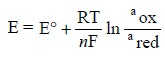Home | | Pharmaceutical Drug Analysis | Amperometric Methods

# Amperometric Methods

An amperometric method or amperometry is concerned with the measurement of current under a constant applied voltage ; and under such experimental parameters the concentration of the ‘analyte’ exclusively determines the quantum and magnitude of the current.

AMPEROMETRIC METHODS

## INTRODUCTION

An amperometric method or amperometry is concerned with the measurement of current under a constant applied voltage ; and under such experimental parameters the concentration of the ‘analyte’ exclusively determines the quantum and magnitude of the current. Hence, these measurements may be employed effectively to record the alteration in concentration of an ion in question in the course of a titration, and ultimately the end-point is established. This specific process is commonly referred to as amperometric method or amperometry.

In this particular case, the total current flowing shall remain almost equal to the current carried by the ions that undergoes equal electrolytic migration together with the current caused on account of the diffusion of the ions. Thus, we have :

I = Id + Im

where   I = Total current,

Id = Diffusion current, and

Im = Migration current.

An awkward situation arises when dealing with a dilute solution where it has been observed that the depletion of the electrode layer ultimately leads to an enhancement of the resistance of the solution and thereby affecting subsequently an alteration in the Ohm’s Law potential drop (I × R) in the cell. This ulti-mately gives rise to a doubtful observed potential operative at the electrode. In order to overcome this serious anomaly, it is a normal practice to add an excess of an indifferent electrolyte to the system, such as : 0.1 M KCl, which renders the solution to remain stable at a low and constant resistance, whereas the migration current (Im) of the species under examination almost vanishes i.e., I = Id.

The ion under investigation, whose rate of diffusion at the electrode surface is governed by Fick’s Law represented as under :where, D = Diffusion coefficient,

C = Concentration,

t = Time, and

x = Distance from the electrode surface.

Thus, the potential of the electrode is controlled and monitored by the Nernst Equation as shown below:### Salient Features of Amperometric Methods :

The various salient features of amperometric titrations are enumerated below :

(a) It is less dependent upon the characteristics of the electrode,

(b) It is quite independent of the nature and type of the supporting electrolyte,

(c) It does not require a constant temperature in the course of a titration but it should not necessarily be fixed accurately,

(d) The substance under investigation may not essentially be reactive at the electrode ; whereas either a reactive reagent or a product is just sufficient for a successful amperometric titration, and

(e) The amperometric method is inherently more accurate and precise, and therefore, has an edge as compared to the polarographic method.

Study Material, Lecturing Notes, Assignment, Reference, Wiki description explanation, brief detail
Pharmaceutical Drug Analysis: Amperometric Methods : Amperometric Methods |